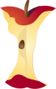Representing Whole Numbers on a Numbers LinePrintable Worksheets And Lessons

• Step-by-step Lesson- Represent the addition and subtraction equations on the numbers line.

• Guided Lesson - We have you start to right your own problems based on the movement of lines on the numbers line.

• Guided Lesson Explanation - The numbers lines are drawn out for you and we ask you to follow the path to the equations.

• Practice Worksheet - Find the sum or difference and then plot it on the numbers line.

• 5 Practice Worksheets - We work on addition and subtraction with the help of a visual numbers line.

• Matching Worksheet - Match the movement of the line on the numbers line and the equation that it should represent.(Click Here to Upgrade)

Homework Sheets

Convert the equation to a numbers line plot.

Practice Worksheets

Write the equation (sum or difference) that you see on the numbers line.

Math Skill Quizzes

We ask you to make sense of the numbers lines by writing them in equation form.

 Quiz 1 Quiz 2 Quiz 3